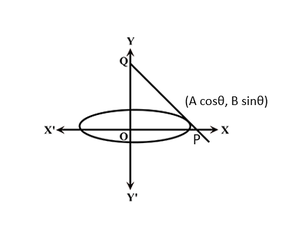GeeksforGeeks App
Open AppBrowser
Continue

# Minimum area of the triangle formed by any tangent to an ellipse with the coordinate axes

Given two integers A and B, representing the length of the semi-major and semi-minor axis of an ellipse with the equation (x2 / A2) + (y2 / B2) = 1, the task is to find the minimum area of the triangle formed by any tangent to the ellipse with the coordinate axes.

Examples:

Input: A = 1, B = 2
Output: 2

Input: A = 2, B = 3
Output: 6

Approach:The idea is based on the observation that the equation of a tangent at coordinate (A * cosθ, B * sinθ) on the ellipse in the figure shown above is:

(x * cosθ / A) + (y * sinθ / B) = 1

The coordinates of P and Q are (A / cosθ, 0) and (0, B / sinθ) respectively.
Area of triangle formed by the tangent to the ellipse and the coordinate axes is given by:

Area of ΔOPQ = 0.5 * base * height = 0.5 * (A / cosθ) * (B / sinθ) = (A * B) / (2 * sinθ * cosθ) = (A * B) / sin2θ

Therefore, Area = (A * B) / sin2θ — (1)

To minimize the area, the value of sin2θ should be maximum possible, i.e. 1.
Therefore, the minimum area possible is A * B.

Below is the implementation of the above approach:

## C++

 `// C++ program for the above approach``#include ``using` `namespace` `std;` `// Function to find the minimum area``// of triangle formed by any tangent``// to ellipse with the coordinate axes``void` `minimumTriangleArea(``int` `a, ``int` `b)``{``    ``// Stores the minimum area``    ``int` `area = a * b;` `    ``// Print the calculated area``    ``cout << area;``}` `// Driver Code``int` `main()``{``    ``int` `a = 1, b = 2;``    ``minimumTriangleArea(a, b);` `    ``return` `0;``}`

## Java

 `// Java program for the above approach``class` `GFG{` `// Function to find the minimum area``// of triangle formed by any tangent``// to ellipse with the coordinate axes``static` `void` `minimumTriangleArea(``int` `a, ``int` `b)``{``    ` `    ``// Stores the minimum area``    ``int` `area = a * b;` `    ``// Print the calculated area``    ``System.out.println(area);``}` `// Driver Code``public` `static` `void` `main(String[] args)``{``    ``int` `a = ``1``, b = ``2``;``    ` `    ``minimumTriangleArea(a, b);``}``}` `// This code is contributed by AnkThon`

## Python3

 `# Python3 program for the above approach` `# Function to find the minimum area``# of triangle formed by any tangent``# to ellipse with the coordinate axes``def` `minimumTriangleArea(a, b):``    ` `    ``# Stores the minimum area``    ``area ``=` `a ``*` `b` `    ``# Print the calculated area``    ``print``(area)` `# Driver Code``a ``=` `1``b ``=` `2` `minimumTriangleArea(a, b)` `# This code is contributed by rohitsingh07052`

## C#

 `// C# program for the above approach``using` `System;` `class` `GFG{``    ` `// Function to find the minimum area``// of triangle formed by any tangent``// to ellipse with the coordinate axes``static` `void` `minimumTriangleArea(``int` `a, ``int` `b)``{``    ` `    ``// Stores the minimum area``    ``int` `area = a * b;` `    ``// Print the calculated area``    ``Console.WriteLine(area);``}` `// Driver Code``public` `static` `void` `Main()``{``    ``int` `a = 1, b = 2;``    ` `    ``minimumTriangleArea(a, b);``}``}` `// This code is contributed by ukasp`

## Javascript

 `// JavaScript program for the above approach` `// Function to find the minimum area``// of triangle formed by any tangent``// to ellipse with the coordinate axes``function` `minimumTriangleArea(a, b)``{``    ` `    ``// Stores the minimum area``    ``var` `area = a * b` `    ``// Print the calculated area``   ``console.log(area)``    ` `}` `// Driver Code``var` `a = 1``var` `b = 2` `minimumTriangleArea(a, b)` `// This code is contributed by AnkThon`

Output

`2`

Time Complexity: O(1)
Auxiliary Space: O(1)

My Personal Notes arrow_drop_up